Mathematics Letters
Volume 2, Issue 6, December 2016, Pages: 42-46

The Geometric Interpretation of Some Mathematical Expressions Containing the Riemann ζ-Function

Yuriy N. Zayko

Department of Applied Informatics, Faculty of Public Administration, The Russian Presidential Academy of National Economy and Public Administration, Saratov Branch, Saratov, RussiaYuriy N. Zayko. The Geometric Interpretation of Some Mathematical Expressions Containing the Riemann ζ-Function. Mathematics Letters. Vol. 2, No. 6, 2016, pp. 42-46. doi: 10.11648/j.ml.20160206.11

Received: October 6, 2016; Accepted: November 10, 2016; Published: December 17, 2016

Abstract: The article discusses some of the mathematical results widely used in practice which contain the Riemann ζ-function, and, at first glance, are in contradiction with common sense. A geometric approach is suggested, based on the concept of the curvature of space, in which is calculated an algorithm that specifies the representation of ζ-function as an infinite diverging series. The analysis is based on the use of Einstein equations to calculate the metric of curved space-time. The solution of the Einstein equations is a metric that has a singularity, like the metric in the vicinity of the black hole. The result can be interpreted in the spirit of a Turing machine that performs the proposed algorithm for calculating the sum of a divergent series.

Keywords: Riemann ζ-Function, Einstein Equations, Metric, Metric Tensor, Energy-Momentum Tensor, Christoffel Symbols, Algorithm, Turing Machine

Contents

1. Introduction

Riemann ζ - function is an element of the mathematical apparatus, widely used in various areas of modern science: mathematics, physics, etc. The results expressed by the ζ-function for humans dealing with them for the first time seem puzzling, although experts perceive them as firmly justified. To such results relates the formula representing ζ (-1) in the form of divergent series used, for example, in the string theory :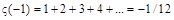.

Although the theory of divergent series is sufficiently developed  nevertheless, it is interesting to try to give a different interpretation of known expressions. Typically, the sum of a divergent series is not computed, but is searching in another way, such as in the case of the known series 1 S =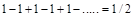. The results presented below can be interpreted as an attempt to find the sums of divergent series by the computational methods. For this purpose, the method of physical analogy was used that is often useful in practice, and which is confirmed in the present case.

2. Physical Approach to Mathematical Problem

Let us formulate a simple physical task - to calculate the distance which is gone by a particle for a time t, moving along a straight line with constant acceleration.

Mathematically, the answer can be represented as a finite sum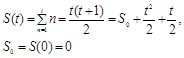(1)

The right side of the formula (1) coincides with the expression for the distance traveled by a particle moving with acceleration a = 1, the initial speed v0 = ½ and the initial position S0 = 0. The time t is assumed discrete and dimensionless. Let us restrict ourselves with only one value of time t = ∞. The answer is known and can be expressed with the help of above mention series: S(∞) = ζ(-1)=-1/12 [1,2]. From a mathematical point of view, it is true, because obtained by analytic continuation of the Riemann ζ-function on the value of the argument u = -1: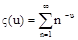(2)

However, it is neither obvious nor clear. The reason is that the value of ζ (-1) is represented by divergent series which does not have the sum in the usual sense, inherent in the concept of the sum of convergent series . While the sum of the convergent series, which is understood as the limit of the partial sums of the series is intuitively clear for divergent series intuition fails to work and you have to attribute "for the word "sum" meaning which is different from the usual" . But even agreeing to this extension of the definition, have to deal with the fact that different sums are ascribed for one and the same divergent series . Other words, the value of the sum depends on the "context". Let’s try to give a geometric interpretation of the process of finding the "sum" of the divergent series and, thus, avoid some contradictions that still exist in this regard.

Recall that the uniformly accelerated motion of the particle requires according to Newton's second law, that a constant force operates on the particle in the direction of its movement. This effect can be achieved by placing at the point x = 0 (the direction of movement of the particle is taken as the axis OX of our frame of reference) an infinite plane coincident with the plane YOZ, having a constant mass density σ. The gravitational potential of this plane is equal to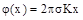, and the force acting on a unit point mass is equal to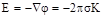and is directed along ОХ, K – is the gravitational constant. The expression for the space-time metric may be found from solving the Einstein's equations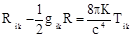(3)

Here R – is the trace of the Ricci tensor Rik: R = Rii, gikis the metric tensor; Tik - is the energy-momentum tensor; с – is the speed of light in vacuum; indices i, k have values 0, 1, 2, 3. Let us write the expression for the interval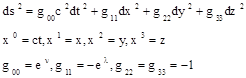(4)

The standard notations for g00 and g11 are used . The solution is very similar to the Schwarzschild solution of the problem of the finding the metric near a point mass . The non-zero Christoffel symbols are the follows :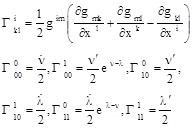(5)

The point means a derivative on ct, prime - on x1=x. Forwhere Tik = 0 the equations (3) can be reduced to the equations Rik=0, which for R00 and R11 lead to a single equation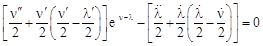(6)

and the equation for R01 is reduced to identity. Assuming λ = -ν, and all time derivatives equal zero, the last equation reduces to the form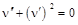(7)

which has a solution eν = C1x+C2, С1,2 – are constants. Their appropriate choice gives the desired solution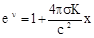(8)

considering the connection of the metric tensor component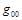and the Newtonian potential φ: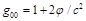. Let write the final form of the interval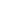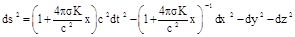(9)

Spatial metric along the direction ОХ (dx=dy=0) is given by the expression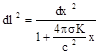(10)

After integration we obtain the relationship between the coordinate x in the system of the remote observer and the distance l, passed by the particle, measured in its rest frame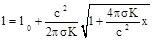(11)

l0- is the integrating constant. From the formula (11) one can obtain the relationship between the lengths of the line segments traversed by the particle, measured in different systems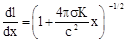(12)

3. The Solution of the Equations of Motion

Let us treat the solutions of the equations of motion of the particle in question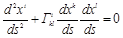(13)

Given the nonzero Christoffel symbols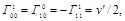and the expression for the derivative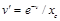, where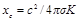we receive the system of two equations(14)

The second equation in is integrated and gives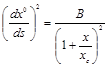(15)

Bis a constant. After substitution into the first equation in it looks as follows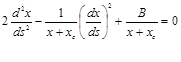(16)

Equation can be integrated once that leads to an expression for the 4-speed (D - is a constant)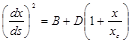(17)

or in another form in variable x0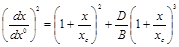(18)

The constants of integration can be found comparing the expression of (18) with corresponding classical result. A classic case corresponds to x << xc. Expanding the right side of the (18) in x we receive the classical limit of (18)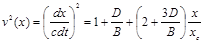(19)

It should be compared with the classical result following from the law of conservation of energy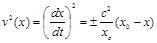(20)

x0 – is the value of х wherein the speed v = 0, signs "±" correspond to the cases x0 > 0 and x > 0 or to the opposite case, taking into account that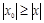. That last case we have to choose due to reasons which will be clear soon. So for the sign"-" we receive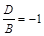(21)

Approach the massive particle to the point xc according to the formula (18) spends an infinite time from the point of view of a distant observer, as is seen in the Fig. 1: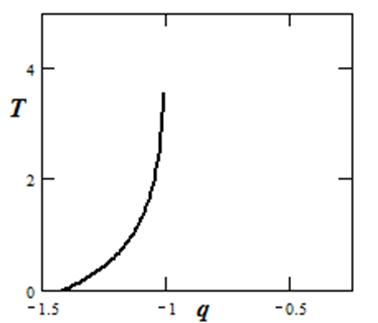Fig. 1. Travelling time of the particle T versus coordinate q = x/xc. Solid line – coordinate time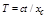in the system of the distant observer received from the solution of (18). Ini- tial point q0 = -1.42 corresponds to the initial speed v0=-0,5.

4. Algorithmic Analysis of the Result

Let us analyze the resulting expression. In case of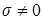the observers in the different systems receives different values. Of particular interest is the case when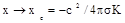, and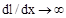, although the value of l stays finite. This means that a moving particle while approaching the specified point xc on either side, in view of a distant observer will never achieves it. This is reminiscent of the behavior of a massive particle in the vicinity of a black hole, from the point of view of the distant observer. The value of xc is an analogue of the event horizon in the Schwarzschild problem.

This makes it possible to remove the contradiction, enclosed in the formula (1). According to calculation of an observer2 moving with the particle, he pass increasingly larger segments of the path, while the remote observer believes he stands still and the resulting distance traveled by him stays finite.

One can say that in the formula (1), the right side is a record of calculation algorithm which is performing by an observer or by a calculation device in its rest frame. The steps of the algorithm description look as follows:

I. Take a segment of length 1.

II. Take a segment of length 2 and attach it to the first segment.

III. Follow this procedure infinitely, providing that length of the subsequent segment is greater the length of preceding one by 1.

IV. Measure the length of the resulting segment.

The final measurement is performed by the remote observer or device. From the standpoint of general relativity their results differ as described above, which explains the contradiction of the formula (1). The use of such "physical" approach implies that the numbers in the formula (1) are regarded as coordinate values in some different frames of references (coordinate maps) applied to the real numbers axis.

5. Calculation the Sum of the Divergent Series

As was mentioned earlier the sums of the divergent series in practice are not calculated but is found from the considerations of another kind . Nevertheless we’ll try to calculate the sum S(∞) (1) with the help of formulas derived above. To this end, using the formula (18) we try to take into account the initial values for the acceleration a0 = a(0)=1, the velocity v0 = v(0) = ½ and the position S0 = S(0) = 0.

It appears that the condition for the velocity cannot be satisfied for real x as seen from the Fig. 2Fig. 2. Velocity of the particle (18) in the system of the distant observer versus coordinate;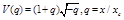.

Thus we slightly change our task and instead searching of the sum S(∞) (1) we will find an another sum S1(∞), where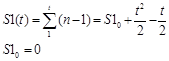(22)

It is obviously that S(∞)=S1(∞) +ζ(0)=S1(∞)-0,5 . The initial values for the S1 are just the same as for the S except the value for the initial speed which looks as follows: v0 = -½, and easily can be satisfied what gives the value for the q0 =-1,42.

The condition S10 = 0 means that the initial point should be placed at the point q0 = -1,42. Then the distance which has been travelled by a massive particle before its stop at point qc = x/xc = - 1 is equal to -1 – (-1,42) - 0,5 = -0,08 from the point of view of the distant observer. This coincides with the exact value for ζ(-1)=-0,0833(3) up to two decimal places, which is due to the finite precision of calculations (10-3).

6. Analysis of the Equation (6)

The described method can be used to find the sums of other divergent series related to the ζ-function. Consider the formula for the trivial zeros of the ζ-function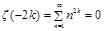(23)

where k – is a positive integer . To apply the method described above, one should consider the motion of the calculating device along the OX-axis with the alternating acceleration. This, obviously, requires finding other solutions to the equation (6). Put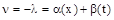. Equation (6) then reduces to the system of two equations (m is a parameter of the variables separation)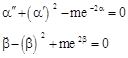(24)

Their solutions look as follows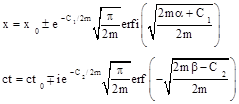(25)

where C1,2 – are constants, and erf и erfi – probability integrals of a real and imaginary argument .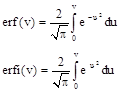(26)

7. Conclusions

The result obtained can be interpreted in the spirit of the theory of relativity of A. Einstein if we treat the calculation as a physical process implemented by material bodies and displayed on the axis of real numbers, which serves as a space of one dimension. The transformation (11) make it possible to explain the results of the calculations with the Riemann ζ-function mentioned above and eliminate their contradiction with common sense.

Riemann ζ- function for more than a century, is the focus of mathematicians all over the world in connection with attempts to prove the famous Riemann hypothesis  concerning its non-trivial zeroes. Numerous attempts both numerical  and theoretical [10,11,12] were made in order to prove or reject the Riemann hypothesis.

The present work could shed new light to this problem.

The used above physical objects can be interpreted in the spirit of a Turing machine that performs the above proposed algorithm, assuming the read-write head has a mass and moving with the variable speed along the resting tape on which the result will be written and which is identified as the system of distant observer.

An overview of the different versions of the relativistic Turing machine is given in the paper .

From the physical point of view, the result received above can be considered as yet another confirmation of the general theory of relativity, along with known experimental confirmations .

Acknowledgments

The author expresses his thanks to prof. Tuyen Truong for his interest in this work and discussions.

References

1. Zwiebach B. A First Course in String Theory, 2-nd Ed., MIT.- 2009.
2. Janke E., Emde F, Lösch F., Tafeln Höherer Funktionen, B.G. Teubner Verlagsgeselschaft, Stuttgart, 1960.
3. Hardy G.H. Divergent series.-Oxford, 1949.
4. Eiler L. Differential Calculation.- Academy Pub., St. Petersburg.- 1755.
5. Landau L.D., Lifshitz E.M., The Classical Theory of Fields. Vol. 2 (4th ed.). Butterworth-Heinemann, 1975.
6. Maxwell’s Demon 2. Entropy, Classical and Quantum Information, Computing. Ed. by Leff H.S., and Rex A.F., IoP Publishing, 2003.
7. Prudnikov A. P., Brychkov Yu. A., and Marichev O. I., Integrals and Series, vols. 1–3; Gordon and Breach, New York, 1986, 1986, 1989.
8. Derbyshire J., Prime obsession. Bernhard Riemann and the Greatest Unsolved Problem in Mathematics, Joseph Henry Press, Washington, D.C., 2003.
9. ZetaGrid Homepage: http://www.wstein.org/simuw/misc/ zeta_grid.html
10. Pei-Chu Hu and Bao Qin Li. A Connection between the Riemann Hypothesis and Uniqueness of the Riemann zeta function, arXiv:1610.01583v1 [math.NT] 5 Oct 2016.
11. McPhedran R.C., Zeros of Lattice Sums: 3. Reduction of the Generalised Riemann Hypothesis to Specific Geometries, arXiv:1610.07932v1 [math-ph] 22 Oct 2016.
12. May M. P., On the Location of the Non-Trivial Zeros of the RH via Extended Analytic Continuation, arXiv:1608.08082v3 [math.GM] 16 Sep 2016.
13. Andr´eka H., N´emeti I., N´emeti P. General relativistic hypercomputing and foundation of mathematics, Natural Computing, 2009, V. 8, № 3, pp 499–516.
14. Weinberg S., Gravitation and Cosmology. Principles and Applications of the General Theory of Relativity, MTI, John Villey & Sons, NY, 1972.

Footnotes

 This result is received from the obvious relation S = 1 - S

 The terminology adopted in physics is used to describe the activity on the measurement of some quantity. So, as has often been noted  between the processes of measurement and calculation is no fundamental difference, there is reason a person (computing device) that calculates algorithm to name him (it) an observer.

 Contents 1. 2. 3. 4. 5. 6. 7.
Article ToolsAbstractPDF(284K)